# JEE Main 2020 Physics Paper With Solutions Sept 4 (Shift 2)

The solutions of JEE Main 2020 Physics (Shift 2-Sept 4th) are available on this page. The IIT aspirants will find it very informative and helpful. Practising these questions will help them in cracking the examination. The candidates should refer to these questions to understand the pattern of the question paper, weightage given to each concept, etc. Click on the link below to download the Physics 2020 Shift 2 paper.

### September 4 Shift 2 - Physics

1. A circular coil has moment of inertia 0.8 kg m2 around any diameter and is carrying current to produce a magnetic moment of 20 Am2. The coil is kept initially in a vertical position and it can rotate freely around a horizontal diameter. When a uniform magnetic field of 4 T is applied along the vertical, it starts rotating around its horizontal diameter. The angular speed the coil acquires after rotating by 60° will be:

1. 1) 10 π rad s–1
2. 2) 20 rad s–1
3. 3) 20 π rad s–1
4. 4) 10 rad s–1

Solution:

By energy conservation

Ui+ Kf = Ui+ Kf

-MBcos600 +0 = -MBcos00+ (1/2)Iω2

(-MB/2) + MB = (1/2)Iω2

$$\omega =\sqrt{\frac{MB}{I}}=\sqrt{\frac{20\times 4}{0.8}}=\sqrt{100}=10rad/s$$

2. A person pushes a box on a rough horizontal platform surface. He applies a force of 200 N over a distance of 15 m. Thereafter, he gets progressively tired and his applied force reduces linearly with distance to 100 N. The total distance through which the box has been moved is 30 m. What is the work done by the person during the total movement of the box?

1. 1) 5690 J
2. 2) 5250 J
3. 3) 2780 J
4. 4) 3280 J

Solution: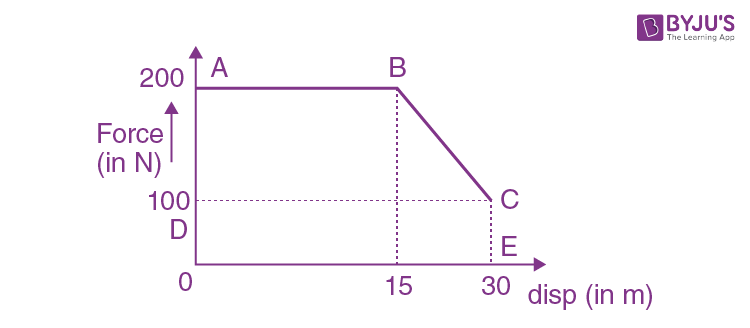Work done = area of ABCEO

= area of trap. ABCD + area of rect. ODCE

= (1/2)x 45x100+100x30=5250J

3. Match the thermodynamic processes taking place in a system with the correct conditions. In the table: ∆Q is the heat supplied, W is the work done and U is change in internal energy of the system.

Process - Condition

(I) Adiabatic - (1) ∆W = 0

(II) Isothermal - (2) ∆ Q = 0

(III) Isochoric - (3) ∆U ≠0, ∆W ≠ 0, ∆Q ≠ 0

(IV) Isobaric - (4) ∆U = 0

1. 1) (I) - (1), (II) - (1), (III) - (2), (IV) - (3)
2. 2) (I) - (1), (II) - (2), (III) - (4), (IV) - (4)
3. 3) (I) - (2), (II) - (4), (III) - (1), (IV) - (3)
4. 4) (I) - (2), (II) - (1), (III) - (4), (IV) - (3)

Solution:

adiabatic, Q = 0

Isothermal, U = 0

Isochoric,ʃpdv = 0

W 0

Isobaric, ∆U ≠0, ∆W ≠ 0, ∆Q ≠ 0

4. The driver of a bus approaching a big wall notices that the frequency of his bus’s horn changes from 420 Hz to 490 Hz when he hears it after it gets reflected from the wall. Find the speed of the bus if speed of the sound is 330 ms–1.

1. 1) 81 kmh–1
2. 2) 91 kmh–1
3. 3) 71 kmh–1
4. 4) 61 kmh–1

Solution: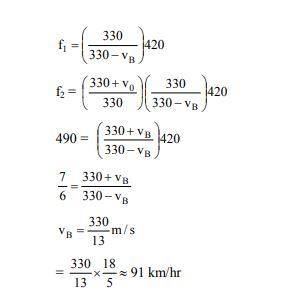5. A small ball of mass m is thrown upward with velocity u from the ground. The ball experiences a resistive force mkv2 where v is its speed. The maximum height attained by the ball is:

1. 1)
$$\frac{1}{k}tan^{-1}\frac{ku^{2}}{2g}$$
2. 2)
$$\frac{1}{2k}In\left [1+\frac{ku^{2}}{g} \right ]$$
3. 3)
$$\frac{1}{2k}In\left [1+\frac{ku^{2}}{2g} \right ]$$
4. 4)
$$\frac{1}{2k}tan^{-1}\left [\frac{ku^{2}}{g} \right ]$$

Solution: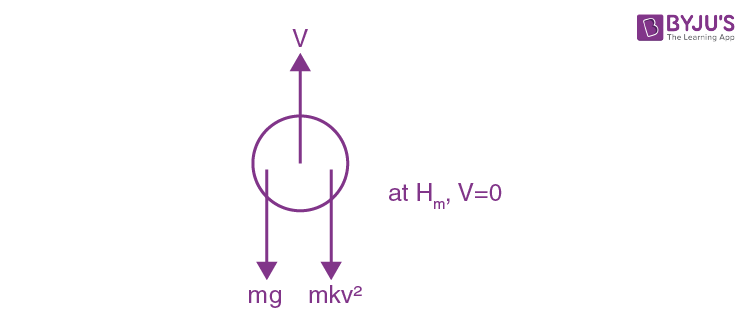$$H_{max}=\frac{1}{2k}ln\left [ \frac{g+ku^{2}}{g} \right ]$$

$$H_{max}=\frac{1}{2k}ln\left [1+ \frac{ku^{2}}{g} \right ]$$

6. Consider two uniform discs of the same thickness and different radii R1= R and R2 =αR made of the same material. If the ratio of their moments of inertia I1 and I2, respectively, about their axes is I1: I2 = 1 : 16 then the value of α is

1. 1) √2
2. 2) 2
3. 3) 2√2
4. 4) 4

Solution: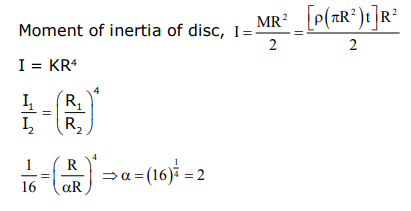7. A series L-R circuit is connected to a battery of emf V. If the circuit is switched on at t =0, then the time at which the energy stored in the inductor reaches (1/n) times of its maximum value, is:

1. 1)
$$\frac{L}{R}In\left [ \frac{\sqrt{n}}{\sqrt{n}+1} \right ]$$
2. 2)
$$\frac{L}{R}In\left [ \frac{\sqrt{n}}{\sqrt{n}-1} \right ]$$
3. 3)
$$\frac{L}{R}In\left [ \frac{\sqrt{n}+1}{\sqrt{n}-1} \right ]$$
4. 4)
$$\frac{L}{R}In\left [ \frac{\sqrt{n}-1}{\sqrt{n}} \right ]$$

Solution: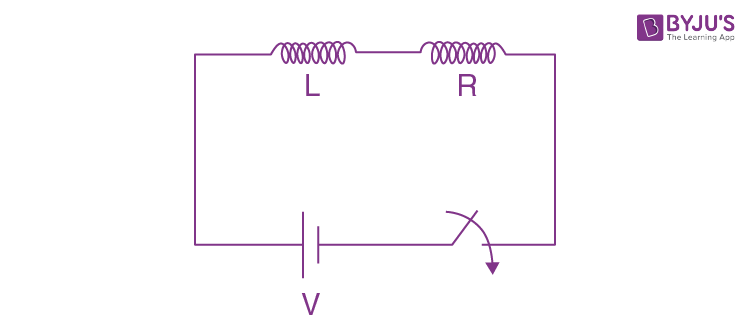P.E. in inductor

$$U=\frac{1}{2}LI^{2}$$

$$U\propto I^{2}$$

$$\frac{U}{U_{0}}=\left [ \frac{I}{I_{0}} \right ]^{2}$$

$$\frac{1}{n}=\left [ \frac{I}{I_{0}} \right ]^{2}$$

$$I= \frac{I_{0}}{\sqrt{n}}$$

$$I= I_{0}(1-e^{-\frac{R}{L}t})$$

$$\frac{I_{0}}{\sqrt{n}}= I_{0}(1-e^{-\frac{R}{L}t})$$

taking In & solving we get,

$$t = \frac{L}{R}ln\left [ \frac{\sqrt{n}}{\sqrt{n}-1} \right ]$$

8. The electric field of a plane electromagnetic wave is given by

$$\vec{E}=E_{0}(\hat{x}+\hat{y})sin(kz-\omega t)$$
. Its magnetic field will be given by:

1. 1)
$$\frac{E_{0}}{c}(\hat{x}+\hat{y})sin(kz-\omega t)$$
2. 2)
$$\frac{E_{0}}{c}(\hat{x}-\hat{y})sin(kz-\omega t)$$
3. 3)
$$\frac{E_{0}}{c}(\hat{x}-\hat{y})cos(kz-\omega t)$$
4. 4)
$$\frac{E_{0}}{c}(-\hat{x}+\hat{y})sin(kz-\omega t)$$

Solution:

$$\vec{E}\times \vec{B}$$
should be in direction of
$$\vec{v}$$

$$\vec{B} = \frac{E_{0}}{c}(-\hat{x}+\hat{y})sin(kz-\omega t)$$

9. A cube of metal is subjected to a hydrostatic pressure of 4 GPa. The percentage change in the length of the side of the cube is close to: (Given bulk modulus of metal, B = 8 × 1010 Pa)

1. 1) 0.6
2. 2) 20
3. 3) 1.67
4. 4) 5

Solution:

$$(-)\frac{\Delta P}{\frac{\Delta V}{V}}=B$$

$$\Delta P=\left | {\frac{\Delta V}{V}} \right |.B$$

$$={\frac{3\Delta L}{L}}\times B$$

Therefore,

$${\frac{\Delta L}{L}}=\frac{\Delta P}{3B}$$

$$\frac{4\times 10^{9}}{3\times8 \times10^{10}} = \frac{1}{60}$$

Therefore, % we get,

$${\frac{\Delta L}{L}}\times 100%$$

Putting values we get = 1.67

10. A paramagnetic sample shows a net magnetisation of 6 A/m when it is placed in an external magnetic field of 0.4 T at a temperature of 4 K. When the sample is placed in an external magnetic field of 0.3 T at a temperature of 24 K, then the magnetisation will be:

1. 1) 4 A/m
2. 2) 1 A/m
3. 3) 0.75 A/m
4. 4) 2.25 A/m

Solution:

$$M = \frac{CB_{ext}}{T}$$

$$6 = \frac{C\times 0.4}{4}$$

$$\Rightarrow C=60$$

Therefore,

$$M = \frac{60\times 0.3}{24}=0.75 A/m$$

11. A body is moving in a low circular orbit about a planet of mass M and radius R. The radius of the orbit can be taken to be R itself. Then the ratio of the speed of this body in the orbit to the escape velocity from the planet is:

1. 1) 2
2. 2) √2
3. 3) 1
4. 4)1/√2

Solution:

1.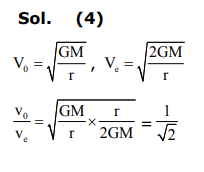12. A particle of charge q and mass m is subjected to an electric field E = E0 (1 – ax2) in the x-direction, where a and E0 are constants. Initially the particle was at rest at x =0. Other than the initial position the kinetic energy of the particle becomes zero when the distance of the particle from the origin is:

1. 1)
$$\sqrt{\frac{2}{a}}$$
2. 2) a
3. 3)
$$\sqrt{\frac{3}{a}}$$
4. 4)
$$\sqrt{\frac{1}{a}}$$

Solution:

W = ∆KE

$$\int_{0}^{x}Fdx = 0$$

$$\int_{0}^{x}qEdx = 0$$

$$q \int_{0}^{x} E_{0}\left(1-a x^{2}\right) d x=0$$

$$q E_{0}\left[\int_{0}^{x} d x-a \int_{0}^{x} x^{2} d x\right]=0$$

$$q E_{0}\left[x-\frac{a x^{3}}{3}\right]=0$$

$$x\left(1-\frac{a x^{2}}{3}\right)=0$$

X = 0,

$$\left(1-\frac{a x^{2}}{3}\right)=0$$

$$\left(\frac{a x^{2}}{3}\right)=1$$

$$x = \sqrt{\frac{3}{a}}$$

13. A capacitor C is fully charged with voltage V0. After disconnecting the voltage source, it is connected in parallel with another uncharged capacitor of capacitance C/2. The energy loss in the process after the charge is distributed between the two capacitors is:

1. 1)
$$\frac{1}{2}CV_{0}^{2}$$
2. 2)
$$\frac{1}{4}CV_{0}^{2}$$
3. 3)
$$\frac{1}{3}CV_{0}^{2}$$
4. 4)
$$\frac{1}{6}CV_{0}^{2}$$

Solution:$$v_{f}=\frac{C V_{0}}{3 \frac{C}{2}}=\frac{2 V_{0}}{3}$$

$$u_{i}=\frac{1}{2} C V_{0}^{2}$$

$$u_{f}=\frac{1}{2}\left(\frac{3 C}{2}\right) \frac{4 V_{0}^{2}}{9}=\frac{C V_{0}^{2}}{3}$$

$$u_{i}-u_{f}=\frac{1}{2} C V_{0}^{2}-\frac{C V_{0}^{2}}{3}$$

$$=C V_{0}^{2}\left(\frac{1}{2}-\frac{1}{3}\right)=\frac{C V_{0}^{2}}{6}$$

14. Find the Binding energy per nucleon for 12050 Sn. Mass of proton mp = 1.00783 U, mass of neutron mn = 1.00867 U and mass of tin nucleus mSn = 119.902199 U. (take 1U = 931 MeV)

1. 1) 8.0 MeV
2. 2) 9.0 MeV
3. 3) 7.5 MeV
4. 4) 8.5 MeV

Solution:

B.E = ∆mc2

=∆ m x 931

∆m = (50 x 1.00783)+(70 x 1.00867)- (119.902199)

= (120.9984 - 119.902199)U

= 1.0962 U

BE = (1.0962 x 931) = 1020.5622MeV

BE per nucleon ≈1020.5622/120 = 8.5 Mev

15. The value of current i1 flowing from A to C in the circuit diagram is: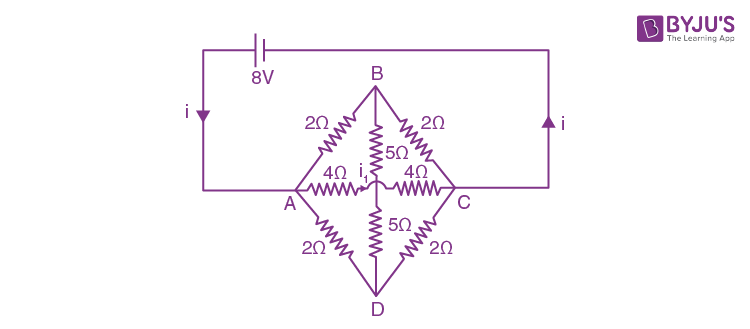1. 1) 4 A
2. 2) 5 A
3. 3) 2 A
4. 4) 1 A

Solution: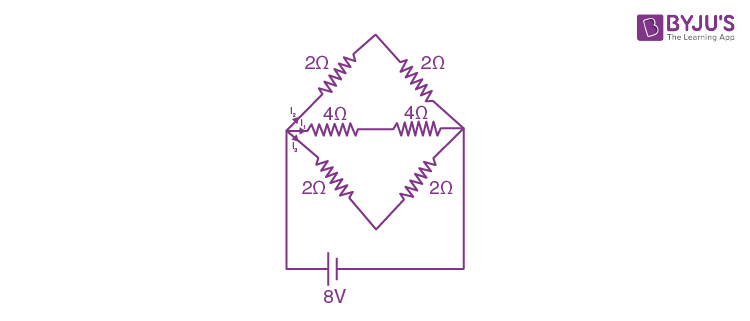16. Two identical cylindrical vessels are kept on the ground and each contain the same liquid of density d. The area of the base of both vessels is S but the height of liquid in one vessel is x1 and in the other, x2. When both cylinders are connected through a pipe of negligible volume very close to the bottom, the liquid flows from one vessel to the other until it comes to equilibrium at a new height. The change in energy of the system in the process is:

1. 1) gdS (x2 + x1)2
2. 2) gdS (x22 + x12)
3. 3) 1/4gdS(x2 – x1)2
4. 4) 3/4gdS(x2 – x1)2

Solution: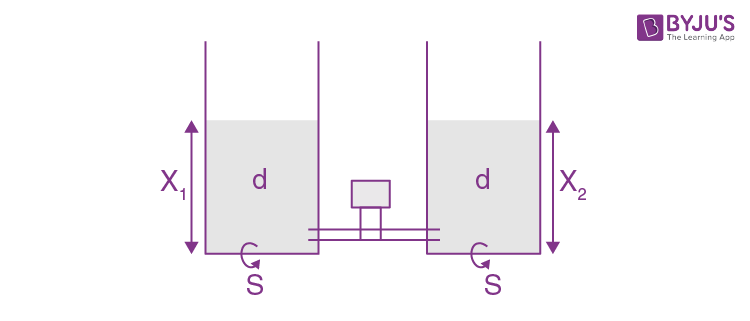$$dSg\left [ \frac{x_{1}^{2}}{2} + \frac{x_{2}^{2}}{2} - x_{1}x_{2}\right ]$$

$$\frac{dSg}{4} (x_{1} - x_{2})^{2}$$

17. A quantity x is given by (IFv2/WL4) in terms of moment of inertia I, force F, velocity v, work W and Length L. The dimensional formula for x is same as that of:

1. 1) coefficient of viscosity
2. 2) energy density
3. 3) force constant
4. 4) planck’s constant

Solution:

$$[x]=\frac{I F v^{2}}{W L^{4}}=\frac{\left(M^{\prime} L^{2}\right)\left(M L T^{-2}\right)\left(L T^{-1}\right)^{2}}{\left(M L^{2} T^{-2}\right) L^{4}}$$

= ML–1T–2 = Energy density

18. For a uniform rectangular sheet shown in the figure, the ratio of moments of inertia about the axes perpendicular to the sheet and passing through O (the centre of mass) and O’ (corner point) is: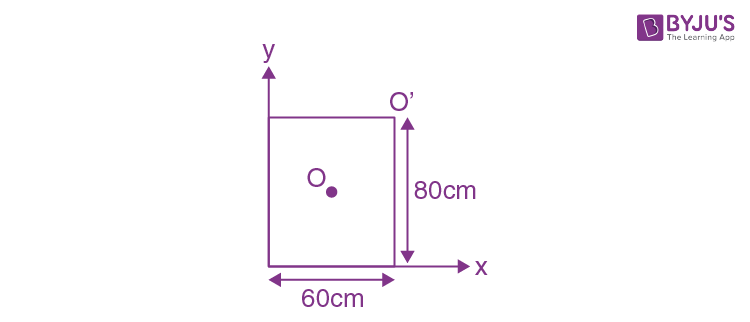1. 1) 1/2
2. 2) 2/3
3. 3) 1/4
4. 4) 1/8

Solution: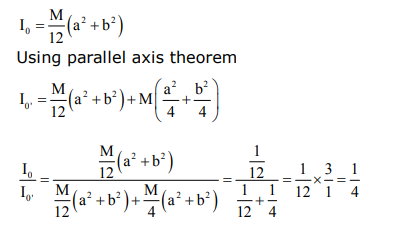19. Identify the operation performed by the circuit given below:1. 1) NOT
2. 2) OR
3. 3) AND
4. 4) NAND

Solution: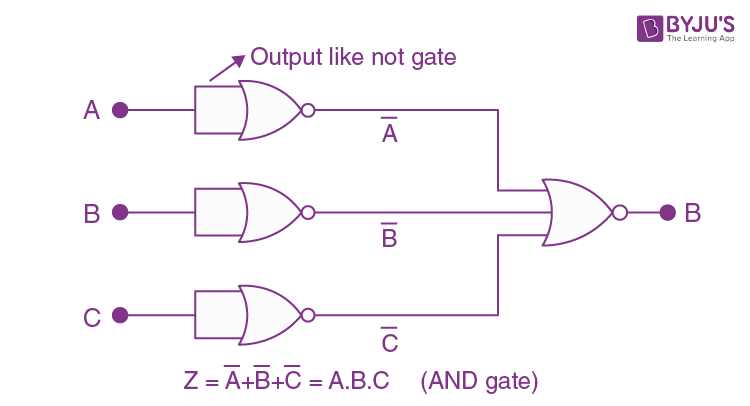20. In a photoelectric effect experiment, the graph of stopping potential V versus reciprocal of wavelength obtained is shown in the figure. As the intensity of incident radiation is increased: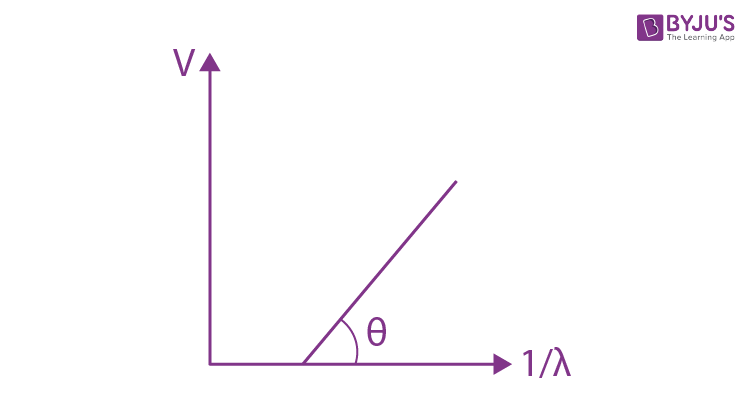1. 1) Straight line shifts to right
2. 2) Straight line shifts to left
3. 3) Slope of the straight line get more steep
4. 4) Graph does not change

Solution:

ev = hν –w (w = work function)

v = (hν –w)/ e

as (h/e) & (w /e) constant.

Therefore no change in graph.

21. The speed verses time graph for a particle is shown in the figure. The distance travelled (in m) by the particle during the time interval t = 0 to t = 5 s will be________.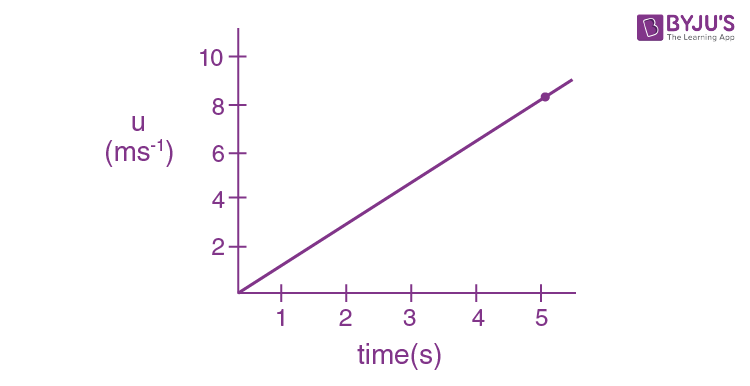Solution:

Distance = Area under speed – time graph

= (1/2) x 8 x 5 = 20m

22. Four resistances 40 Ω, 60 Ω, 90 Ω and 110 Ω make the arms of a quadrilateral ABCD. Across AC is a battery of emf 40 V and internal resistance negligible. The potential difference across BD in V is _______.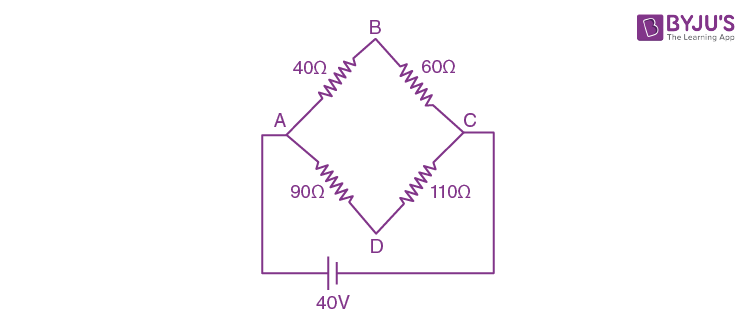Solution: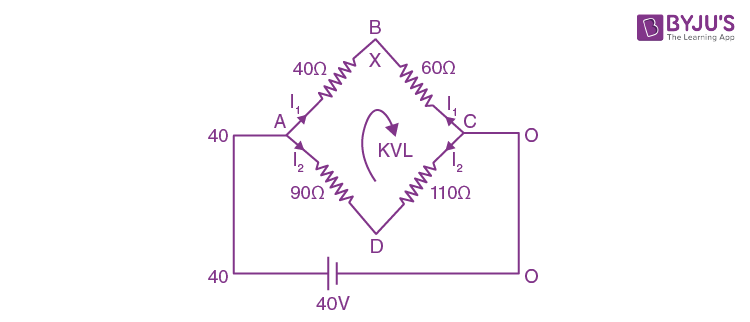$$V_{B}-\left [ \frac{40}{100}\times 60 \right ]+\left [ 110\times \frac{40}{200} \right ]-V_{D}=0$$

VB – VD = 24 -22

= 2V

23. The change in the magnitude of the volume of an ideal gas when a small additional pressure ∆P is applied at a constant temperature, is the same as the change when the temperature is reduced by a small quantity ∆T at constant pressure. The initial temperature and pressure of the gas were 300 K and 2 atm. respectively. If │∆T│= C│∆ P│ then value of C in (K/atm.) is _________.

Solution:

1st case

PV = nRT

PdV + VdP = 0

P∆V + V∆P = 0 (∆V = (-∆P/P)v)

2nd case

PV = (–nRT/P) (∆V = (-nRT /P))

$$\frac{-\Delta P}{P}V=\frac{-nR\Delta T}{P}\Rightarrow \Delta T=\Delta P\frac{v}{nR}$$

$$\Rightarrow \frac{\Delta T}{\Delta P}=\frac{V}{nR}$$

Now, given │∆T│= C│∆ P│

$$C=\frac{\Delta T}{\Delta P}=\frac{V}{nR}$$

$$C=\frac{ T}{P}=\frac{300}{2}=150$$

24. Orange light of wavelength 6000×10–10 m illuminates a single slit of width 0.6 × 10–4 m. The maximum possible number of diffraction minima produced on both sides of the central maximum is ___________.

Solution:

For minima

d sin θ = nλ

or sin θ = nλ/d

maximum value of sin θ is 1

Therefore,

(nλ/d) ≤ 1

n ≤ d/λ

$$n\leq \frac{0.6 \times 10^{-4}}{6000\times 10^{-10}}$$

$$n\leq 100$$

for both sides 100 +100 = 200

25. The distance between an object and a screen is 100 cm. A lens can produce real image of the object on the screen for two different positions between the screen and the object. The distance between these two positions is 40 cm. If the power of the lens is close to (N/100)D where N is an integer, the value of N is _________.

Solution:

$$f=\frac{D^{2}-d^{2}}{4D}=\frac{100^{2}-40^{2}}{400}$$

$$=\frac{10000-1600}{400}$$

$$=\frac{100-16}{4}=\frac{84}{4}=21$$

$$p=\frac{1}{f}=\frac{1}{21}=\frac{1}{21}\times \frac{100}{100}=\left [ \frac{4.76}{100} \right ]=\frac{N}{100}$$

Therefore, N ≈5

### JEE Main 2020 Physics Paper With Solutions Shift 2 September 4